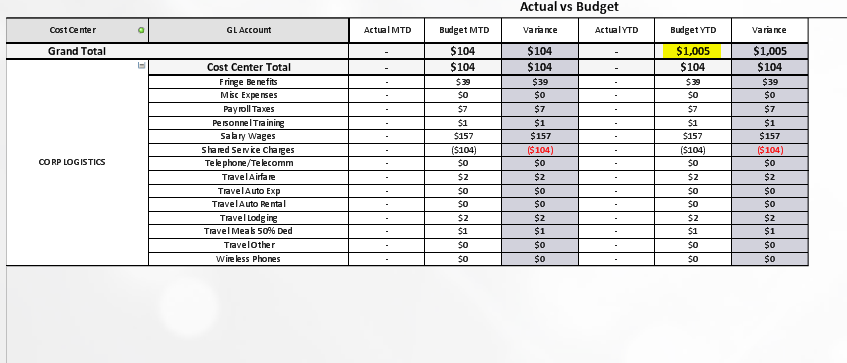# QlikView App Dev

Discussion Board for collaboration related to QlikView App Development.

Announcements
Join us at the Cloud Data and Analytics Tour! REGISTER TODAY
cancel
Showing results for
Search instead for
Did you mean:Contributor

## Pivot Table Totals

I have a pivot table that calculates MTD and YTD. The problem is that the expression total (for YTD) does not respond to selections. For example, when I select January the Grand totals for MTD and YTD are calculating correctly, but when I select a cost center, the MTD will adjust, but the YTD will be frozen. See screen shots below.

The YTD formula I am using is sum( {1<[CLMonthID] = {"<=\$(vCLMonthID)"} >}  [CL B2017])2 RepliesContributor III

Try changing the formula into:

sum( {\$<[CLMonthID] = {"<=\$(vCLMonthID)"} >}  [CL B2017])MVP

Why do you have 1 in your set expression?

Sum({1<[CLMonthID] = {"<=\$(vCLMonthID)"}>}  [CL B2017])

May be try without it?

Sum({<[CLMonthID] = {"<=\$(vCLMonthID)"}>}  [CL B2017])

or allow for selection selection in Cost Center

Sum({1<[CLMonthID] = {"<=\$(vCLMonthID)"}, [Cost Center] = \$::[Cost Center]>}  [CL B2017])